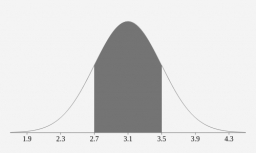# The probability

The probability that a life bulb will have a lifetime of more than 682 hours is 0.9788. The probability that a bulb will have a lifetime of more than 703 hours is 0.0051. Find the probability that a bulb will last for more than 648 hours.

p =  1

### Step-by-step explanation:Did you find an error or inaccuracy? Feel free to write us. Thank you!

Tips for related online calculators
Looking for help with calculating arithmetic mean?
Looking for a statistical calculator?
Looking for a standard deviation calculator?
Need help calculating sum, simplifying, or multiplying fractions? Try our fraction calculator.
Do you have a linear equation or system of equations and looking for its solution? Or do you have a quadratic equation?
Would you like to compute the count of combinations?

#### Grade of the word problem:

We encourage you to watch this tutorial video on this math problem: